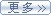#### 密码如上图，文字运动之后并没有马上停止，而是由于惯性进行了回弹。其实这种动画只要你会添加简单的关键帧，加上一段复制的代码就可以实现。在AE合成中，输入文字“AEPark”之后，为文字图层制作位移动画，两个关键帧就足够

以下是一段惯性表达式代码（或叫弹性表达式），把它复制下来。

`//惯性表达式 `
`amp=0.15;`
`freq=1.5; `
`decay=1; `
`n=0; `
`if(numKeys>0){ n=nearestKey(time).index; `
`if(key(n).time>time){ n--; }} `
`if(n==0){t=0; }else{ t=time-key(n).time; } `
`if(n>0){ v=velocityAtTime(key(n).time-thisComp.frameDuration/10); `
`value+v*amp*Math.sin(freq*t*2*Math.PI)/Math.exp(decay*t); }else{value}`

在合成中选择“位置”参数，点击“ctrl+alt+=”键(或者“alt+鼠标左键点击位置属性旁边的秒表”)，为位置参数添加表达式。如下图，将空白区域蓝色底的文字删除，将上方复制的代码粘贴进去。